3天内不再提示

#NVIDIA TensorRT网络层详解

## A.1. TensorRT Layers

TensorRT 可以通过融合层来优化性能。

### A.1.1. IActivationLayer

IActivationLayer实现元素激活函数。

rectified Linear Unit (ReLU): B = ReLU(A)
Hyperbolic tangent: B = tanh(A)
“s” shaped curve (sigmoid): B = σ(A)


### A.1.2. IAssertionLayer

Assert输入维度相等可能有助于优化器。例如，如果网络有两个前导维度必须相等的输入，则使用IGatherLayer（或ISliceLayer）提取这些维度，将它们与ElementWiseOperation::kEQUAL进行比较，并将输出馈送到IAssertionLayer让 TensorRT 知道维度必须相等。

### A.1.3. IConcatenationLayer

IConcatenationLayer将多个具有相同非通道大小的张量沿通道维度链接在一起。

m个输入张量$A^i$和通道轴c的数组中传递。

$b_j ={a_j\ \ \ if \ \ j≠c\ \ \ and\ \ \ \sum {m−1\atop i=0}\ a{i\atop c} \ \ otherwise}$

### A.1.4. IConditionLayer

IConditionLayer表示与 Conditional 构造关联的条件边界。它由 TensorRT 在使用IIfConditional::setCondition时创建。 if-conditional 由条件边界层定义。

IConditionLayer只有一个输入，它接受一个布尔张量，该张量控制与此IConditionLayer关联的 Conditional 的评估流。

IConditionLayer的输入必须是布尔标量（零维张量）。

### A.1.5. IConstantLayer

IConstantLayer输出一个张量，其值作为该层的参数提供，从而可以方便地在计算中使用常量。

### A.1.6. IConvolutionLayer

IConvolutionLayer计算带或不带偏差的2D（通道、高度和宽度）卷积或 3D（通道、深度、高度和宽度）卷积。

4D tensor A上计算与 2D filters 的互相关，尺寸为 a ，以生成 4D 张量B ，尺寸为b 。 B的维度取决于A的维度、输出映射的数量m 、内核大小k 、对称填充p 、步幅s 、扩张d和扩张内核大小 $t = 1+d (k−1)$ ，因此高度和宽度相应调整如下：

• $b =[a_0\ \ \ m\ \ \ b_2\ \ \ b_3]$
• $b_2 =⌊ (a_2+2p_0−t_0)/s_0⌋ + 1$
• $b_3 =⌊ (a_3+2p_1−t_1)/s_1⌋ + 1$

g的核权重w和偏置权重x（可选）如下：

• w根据形状 $[m\ a_1/g\ r_0\ r1]$排序
• x的长度为m

• $k_{i, j, {hh}, {ll}}= w_{i, j, h, l}$
• $hh = {0\ \ \ if\ \ \ h = 0, h + d_0 \ \ (h−1) \ \ otherwise}$
• $ll = {0\ \ if\ \ l = 0, l + d_1 (l−1) \ \ otherwise}$

$B_{i, j, k, l}=\sum(C_{i, :, k:kk, l:ll}× K_{j, :, :, :}) +x_j\ \ \ where\ \ kk = k+t_0−1\ \ and\ \ ll = l+t_1−1$

5D tensor A上计算与 3D filter的互相关，以产生尺寸为b的5D 张量B。 B的维度取决于A的维度、输出映射的数量m 、内核大小k 、对称填充p 、步幅s 、扩张d和扩张内核大小$t = 1+d (k−1)$，因此高度和宽度相应调整如下：

• $b =[a_0\ m \ b_2\ b_3\ b_4]$
• $b_2 = (a_2+2p_0−t_0)/s_0+1$
• $b_3 = (a_3+2p_1−t_1)/s_1+1$
• $b_4 = (a_4+2p_2−t_2)/s_1+1$

g的核权重w和偏置权重x（可选）如下：

• w根据形状$[m\ a_1/g\ r_0\ r_1\ r_2]$排序
• x的长度为m

• $k_{i, j, dd, hh, ll}= w_{i, j, d, h, l}$
• $dd = {0\ \ if\ \ d = 0, d + d_0\ \ (d−1)\ \ otherwise}$
• $hh = {0\ if\ \ h = 0, h + d_1\ \ (h−1) \ otherwise}$
• $ll = {0\ \ if\ \ l = 0, l + d_2\ \ (l−1)\ \ otherwise}$

$B_{i, j, d, k, l}=\sum(C_{i, :, d:dd, k:kk, l:ll} × K_{j, :, :, :, :}) +x_j \ \ where\ \ dd = d+t_0−1 , kk = k+t_1−1 , and\ \ ll = l+t_2−1$ .

### A.1.7. IDeconvolutionLayer

IDeconvolutionLayer计算带或不带偏差的2D（通道、高度和宽度）或 3D（通道、深度、高度和宽度）反卷积。

4D tensor A上计算与 2D filter的互相关，尺寸为 a ，以生成 4D 张量B ，尺寸为b 。 B的维度取决于A的维度、输出映射的数量m 、内核大小k 、对称填充p 、步幅s 、扩张d和扩张内核大小$t = 1+d (k−1)$ ，因此高度和宽度相应调整如下：

• $b =[a_0\ m\ b_2\ b_3]$
• $b_2 = (a_2−1)*s_0 + t_0 − 2p_0$
• $b_3 = (a_3−1)*s_1 + t_1 − 2p_1$

g的核权重w和偏置权重x（可选）如下：

• w是根据形状$[a_1/g\ m\ r_0\ r_1]$排序
• x的长度为m

• $k_{i, j, hh, ll}= w_{i, j, h, l}$
• $hh = {0\ \ if\ \ h = 0, h + d_0 (h−1)\ \ otherwise}$
• $ll = {0\ \ if\ \ l = 0, l + d_1 (l−1) \ \ otherwise}$

$B_{i, j, k, l}=\sum{u, v} (C{i, j, k-u, l-v} \ K) +x_j$

5D tensor A上计算与 3D filter 的互相关，以产生尺寸为b的5D 张量B。 B的维度取决于A的维度、输出映射的数量m 、内核大小k 、对称填充p 、步幅s 、扩张d和扩张内核大小$t = 1+d (k−1)$ ，因此高度和宽度相应调整如下：

• $b =[a_0\ m\ b_2\ b_3]$
• $b_2 = (a_2−1)*s_0 + t_0 − 2p_0$
• $b_3 = (a_3−1)*s_1 + t_1 − 2p_1$
• $b_4 = (a_4−1) *s_2 +t_2 −2p_2$

g的核权重w和偏置权重x（可选）如下：

• w是根据形状$[a_1/g\ m\ r_0\ r_1\ r_2]$排序
• x的长度为m

• $k_{i, j, dd, hh, ll}= w_{i, j, d, h, l}$
• $dd = {0 \ \ if\ \ d = 0, d + d_0 (d−1) \ \ otherwise}$
• $hh = {0\ \ if\ \ h = 0, h + d_1 (h−1)\ \ otherwise}$
• $ll = {0\ \ if\ \ l = 0, l + d_2 (l−1) \ \ otherwise}$

$B_{i, j, k, l, m}=\sum{u, v, w} (C{i, j, k-u, l-v, m-w} \ K) +x_j$

2D 或 3D 由输入内核维度的数量决定。对于 2D 反卷积，输入和输出可能有超过 4 个维度；超过 4，所有维度都被视为批量大小的乘数，输入和输出被视为 4D 张量。对于 3D 反卷积，类似于 2D 反卷积，超过 5 的维度被视为批量大小的乘数。如果指定了组并且使用了 INT8 数据类型，则输入和输出组的大小必须是 4 的倍数。

### A.1.8. IDequantizeLayer

DequantizeLayer实现反量化运算符。

IDequantizeLayer层接受带符号的 8 位整数输入张量，并使用配置的比例和零点输入根据以下公式对输入进行反量化：

output = (input - zeroPt) * scale

### A.1.9. IEinsumLayer

IEinsumLayer实现了一个Einsum运算符。

IEinsumLayer根据Einstein求和约定，沿方程参数指定的维度对输入元素进行求和。

• 该等式以与维度相同的顺序为输入中的每个维度指定 ASCII 小写字母，每个输入用逗号分隔。
• 该等式表示为term1,term2…->output-term ，其中每个项对应于一个操作数张量，项内的字符对应于操作数维度。
• 标有相同下标的尺寸必须匹配。
• 一个输入中的重复下标标签采用对角线。
• 跨多个输入重复标签意味着这些轴将成倍增加
• 从输出中省略标签意味着沿这些轴的值将被求和。
• 对于某些输入操作数，输出下标必须至少出现一次，而对于输出则至多出现一次。
• 在隐式模式下，即如果等式不包含-> ，则表达式中出现一次的索引将成为按字母顺序递增的输出的一部分。
• 在显式模式下，可以通过添加箭头 ( -> ) 后跟输出下标来指定输出下标标签来控制输出。例如，ij,jk->ik等价于ij,jk
• 空字符串 (“”) 对标量操作数有效。
• 该等式可能在不同元素（下标、省略号、箭头和逗号）之间包含空格（SPC-0x20）。

TensorRT 不支持Einsum的省略号、对角线运算或两个以上的输入。所有输入必须具有相同的数据类型，并且该数据类型必须是DataType::kFLOATDataType::kHALF

### A.1.10. IElementWiseLayer

IElementWiseLayer也称为Eltwise层，实现了每个元素的操作。

IElementWiseLayer支持以下操作：

Sum: C = A+B
Product: C = A*B
Minimum: C = min(A, B)
Maximum: C = max(A, B)
Subtraction: C = A-B
Division: C = A/B
Power: C = A^B
Floor division : C = floor(A/B)
And : C = A & B
Or : C = A | B
Xor : C = A xor B
Equal : C = (A == B)
Greater : C = A > B
Less: C = A < B


A和B的每个维度的长度必须等于或等于 1。

IElementWiseLayer层可以接受不同的输入数据类型，具体取决于所使用的操作。IElementWiseLayer支持每个操作的以下类型：

| Operation   | DataTypes                    |
| ----------- | ---------------------------- |
| kSUM        | kFLOAT, kHALF, kINT8, kINT32 |
| kPROD       | kFLOAT, kHALF, kINT8, kINT32 |
| kMAX        | kFLOAT, kHALF, kINT8, kINT32 |
| kMIN        | kFLOAT, kHALF, kINT8, kINT32 |
| kSUB        | kFLOAT, kHALF, kINT8, kINT32 |
| kDIV        | kFLOAT, kHALF, kINT8, kINT32 |
| kPOW        | kFLOAT, kHALF, kINT8         |
| kFLOOR\_DIV | kFLOAT, kHALF, kINT8, kINT32 |
| kAND        | kBOOL                        |
| kOR         | kBOOL                        |
| kXOR        | kBOOL                        |
| kEQUAL      | kFLOAT, kHALF, kINT8, kINT32 |
| kGREATER    | kFLOAT, kHALF, kINT8, kINT32 |
| kLESS       | kFLOAT, kHALF, kINT8, kINT32 |


#### A.1.10.1. ElementWise Layer Setup

ElementWise 层用于执行完全连接层提供的功能的第二步。fcbias常数层和矩阵乘法层的输出用作ElementWise层的输入。然后将该层的输出提供给TopK层。下面的代码演示了如何设置图层：

auto fcbias = network->addConstant(Dims2(VOCAB_SIZE, 1), weightMap[FCB_NAME]);
*matrixMultLayer->getOutput(0),
*fcbias->getOutput(0), ElementWiseOperation::kSUM);
addBiasLayer->getOutput(0)->setName("Add Bias output");

### A.1.11. IFillLayer

IFillLayer用于生成具有指定模式的输出张量。

IFillLayer支持以下操作：

• LINSPACE ：Output = alpha(scalar) + beta(different on each axis) * element_index
• RANDOM_UNIFORM ：Output = Random(min = alpha, max = beta)

### A.1.12. IFullyConnectedLayer

IFullyConnectedLayer实现了一个矩阵向量乘积，有或没有偏差。

IFullyConnectedLayer需要三个或更多维度的输入张量A。给定一个尺寸为$a=[a_0 … a_{n−1}]$ , 它首先被重新整形为尺寸为 $a^′=[a_0 … a_{n−4} (a_{n−3}*a_{n−2}*a_{n−1})]$ 将最后三个维度压缩为一个维度。

A必须具有三个或更多维度。

### A.1.13. IGatherLayer

IGatherLayer实现了聚合操作的三种变体。

GatherND模式：IGatherLayerGatherMode ::kND模式使用维度b的索引张量B收集维度a的数据张量A的元素，以产生维度c的输出C。

• If $k = a − n$
• $shape (C) = [g_1, … g_{b−1}]$
• $C (i_1 … i_{n−1}, i_n, … i_{b−1}) = A (i_1 … i_{n−1}, B (i_1, … i_{b−1}))$
• If $k < a − n$
• $shape (C) = [g_1, … g_{b−1}, f_{n+k+1}, … f_a]$
• $C (i_1, … i_{n−1}, i_n, … i_{a−1}, i_a, … i_{a+b-k-1-n}) = A (i_1, … i_{n−1}, B (i_{1, … ib−1}), i_b, … i_{a+b-k-1-n})$
• where $B (i_1, … i_{b−1})$ is a tensor with k dimensions, and $shape (B (i_1, … i_{b−1})) = [i_n, … i_{n+k+1}]$ .
• If k > a − n
• This is a build time error.

GatherElements模式：IGatherLayerGatherMode ::kELEMENT模式使用维度b的索引张量B在指定轴上收集维度a的数据张量A的元素，以产生维度c的输出C。

For each element X of B:
Let J denote a sequence for the subscripts of X
Let K = sequence J with element [axis] replaced by X
C[J] = A[K]

• 索引张量B必须仅包含 INT32 值。
• 如果索引张量中有任何无效的索引元素，则零将存储在输出张量中的适当位置。
• A的轴具有动态长度，则对其使用负索引具有未定义的行为。

Default Gather Mode：适用于隐式批处理模式：

• 不能沿批量大小维度收集元素。
• 数据张量A必须至少包含一个非批量维度。
• 数据张量A必须至少包含轴+ 1 个非批量维度。
• 参数轴是零索引的，从数据张量A的第一个非批量维度开始。

• 数据张量A必须至少包含一维。
• 数据张量A必须至少包含轴+ 1 个维度。
• 参数轴是零索引的，从数据张量A的第一维开始。
• 轴必须大于或等于IGatherLayer::setNbElementWiseDimensions()设置的元素维度数。
• ElementWise 维度的数量只能设置为 0 或 1。
• 如果 ElementWise 维数设置为 1，则行为将类似于隐式批处理模式。前导维度将被视为隐式批处理模式中的批处理维度，这不是收集操作的一部分。例如，数据张量A的维度为[N, C, H, W]，索引张量B的维度为[N, K]。如果nbElementWiseDimensions为 1 并且轴设置为 1，则结果的维度将为[N, K, H, W]。如果nbElementWiseDimensions为 0 并且轴设置为 1，则结果的维度将为[N, N, K, H, W]
• ElementWise 维度支持像IElementWiseLayer这样的广播。

GatherND Mode：

• 数据张量A必须至少包含一维。
• 索引张量B必须至少包含一维。
• A和B的前n 个维度必须相等，其中n是元素维度的数量。
• n必须小于A的维数和B的维数。
• B的最里面的维度应该有一个介于1和a – n之间的值，包括 1 和 a – n 。

GatherElements Mode：

• 数据张量A必须至少包含一维。
• 索引张量B必须至少包含一维。
• 轴必须介于0和-1之间，包括在内。

### A.1.14. IIdentityLayer

IIdentityLayer实现identity操作。

### A.1.15. IIfConditionalBoundaryLayer

IIfConditionalBoundaryLayer是 if 条件相关层的基类，特别是IConditionLayerIIfConditionalOutputLayerIIfConditionalInputLayerif-conditional由条件边界层定义。

IIfConditionalBoundaryLayer类定义了一个虚拟方法getConditional()，它返回一个指向关联IIfConditional的指针。

### A.1.16. IIfConditionalOutputLayer

IfConditionalOutputLayer指定 if 条件的输出。 if-conditional 由条件边界层定义。

if-conditional必须有一个或多个输出，每个输出由IfConditionalOutputLayer的唯一实例定义。IfConditionalOutputLayer正好有两个输入，一个来自真分支（then-branch），一个来自假分支（else-branch）。当 if-conditional 执行IfConditionalOutputLayer时，它会根据与 if-conditional 关联的条件值选择两个输入中的哪一个复制到输出。

### A.1.17. IIfConditionalInputLayer

IfConditionalInputLayer指定 if 条件的输入。if-conditional由条件边界层定义。

IfConditionalInputLayerif-conditional输入的占位符，可由 if-conditional 的一个或两个分支使用。IfConditionalInputLayer划分了 if 条件的输入边界。

### A.1.18. IIteratorLayer

IIteratorLayer使循环能够迭代张量。循环由循环边界层定义。

### A.1.20. ILoopOutputLayer

ILoopOutputLayer指定循环的输出。循环由循环边界层定义。

### A.1.21. ILRNLayer

ILRNLayer实现跨通道本地响应归一化（LRN） 。

A必须具有三个或更多维度。以下列表显示了参数的可能值：

$W ϵ{1, 3, 5, 7, 9, 11, 13, 15}$

$α ϵ[­1 ×10^{20}, 1 ×10^{20}]$

$β ϵ[0.01, 1 ×10^5]$

$k ϵ[1 × 10­^5, 1 ×10^{10}]$

### A.1.22. IMatrixMultiplyLayer

IMatrixMultiplyLayer为一组矩阵实现矩阵乘法。

IMatrixMultiplyLayer计算输入张量A （尺寸为 a ）和B （尺寸为b ）的矩阵乘法，并生成输出张量C （尺寸为c ）。 A 、 B和C都具有相同的秩n≥2。如果n>2，则A 、 B和C被视为矩阵的集合； A和B可以选择转置（转置应用于最后两个维度）。令$A^I$和$B^I$为可选转置后的输入张量，则

$C_{i_0, . . , i_{n-3}, :, :}=A^I_{i_0, . ., i_{n−3}, :, :}*B^I_{i_0, . ., i_{n−3}, :, :}$

$A^I$ 和$B^I$的对应维度$a^I$和$b^I$ ，则 $C_i={max(a_i,b_i)\ \ \ if\ \ \ i 条件和限制 张量A和B必须至少有两个维度，并且在维度的数量上达成一致。每个维度的长度必须相同，假设广播长度为 1 的维度以匹配对应的长度。 #### A.1.22.1. MatrixMultiply Layer Setup 矩阵乘法层用于执行全连接层提供的功能的第一步。如下面的代码所示，需要使用一个常量层，以便可以将全连接权重存储在引擎中。然后将常量和 RNN 层的输出用作矩阵乘法层的输入。 RNN 输出被转置，以便 MatrixMultiply 的维度有效。 weightMap["trt_fcw"] = transposeFCWeights(weightMap[FCW_NAME]); auto fcwts = network->addConstant(Dims2(VOCAB_SIZE, HIDDEN_SIZE), weightMap["trt_fcw"]); auto matrixMultLayer = network->addMatrixMultiply( *fcwts->getOutput(0), false, *rnn->getOutput(0), true); assert(matrixMultLayer != nullptr); matrixMultLayer->getOutput(0)->setName("Matrix Multiplication output");  有关更多信息，请参阅TensorRT API 文档 空张量 将维度为[ m ,0][0, n ]的矩阵相乘会得到维度为[ m , n ]的零矩阵。它是零，因为结果的每个元素都是空乘积集的总和。 IFullyConnectedLayer本质上是一个矩阵乘法，因此适用类似的规则。 ### A.1.23. IParametricReluLayer IParametricReluLayer表示参数ReLU操作，这意味着 leaky ReLU，其中 x < 0 的斜率对于每个元素可能不同。 层描述 用户提供数据张量X和斜率张量S。在每个元素处，如果x ≥ 0，则该层计算y = x，如果x < 0 则计算y = x∙s。可以将斜率张量广播到数据张量的大小，反之亦然。 条件和限制 许多融合不支持参数化 ReLU；因此，性能可能比标准 ReLU 差。 ### A.1.24. IPaddingLayer IPaddingLayer沿两个最里面的维度实现张量的空间零填充。 注意： IPaddingLayer在 TensorRT 8.2 中已弃用，并将在 TensorRT 10.0 中删除。使用ISliceLayer填充张量，支持新的非常量，反映填充模式和钳位，支持动态形状的填充输出。 层描述 IPaddingLayer沿两个最内层维度中的每一个向输入张量A填充零（或修剪边缘），并给出输出张量B 。每个维度上的填充可以是不同的，不对称的，并且可以是正的（导致张量的扩展）或负的（导致修剪）。二维开始和结束处的填充由二维向量x和y指定，分别用于前填充和后填充。 n维a的输入张量A ，输出B将具有n维b使得$b_i={x_0+a_n−2+y_0\ \ \ if\ \ \ i=n−2; x_1+a_n−1+y_1\ \ \ if\ \ \ i=n−1; and\ \ \ a_i\ \ \ otherwise}$。 因此，如果$w_{n−2}

• A必须具有三个或更多维度。
• 填充只能沿两个最里面的维度应用。
• 仅支持零填充。

### A.1.25. IPluginV2Layer

IPluginV2Layer提供了通过对不受支持的层使用自定义实现来扩展 TensorRT 功能的能力。

IPluginV2Layer用于设置和配置插件。有关 API 的更多详细信息，请参阅IPluginV2 API 说明。 TensorRT 还支持插件注册表，它是网络中所有插件的单一注册点。为了向注册表注册插件，请为您的插件实现IPluginV2类和IPluginCreator类。

• volume为零
• 一个或多个步幅为零

int volume = N*C*H*W;
int cStride = H*W;
...
assert(C == volume/N/cStride);

### A.1.26. IPoolingLayer

IPoolingLayer在通道内实现池化。支持的池化类型是maximumaveragemaximum-average blend

• $b =[a_0\ \ a_1… a_{n−3} b_{n−2} b_{n−1}]$
• $b_{n−2} =( a_{n−2}+2p_0+ r_0) /s_0+1$
• $b_{n−1} =( a_{n−1}+2p_1+ r_1) /s_1+1$

PoolingType::kMAX

PoolingType::kAVERAGE

PoolingType::kMAX_AVERAGE_BLEND

• $b =[a_0\ \ a_1… a_{n−4} b_{n−3} b_{n−2}b_{n−1}]$
• $b_{n−3} =( a_{n−3}+2p_0+ r_0) /s_0+1$
• $b_{n−2} =( a_{n−2}+2p_1+ r_1) /s_1+1$
• $b_{n−1} =( a_{n−1}+2p_2+ r_2) /s_2+1$

PoolingType::kMAX

PoolingType::kAVERAGE

PoolingType::kMAX_AVERAGE_BLEND

A.1.27. IQuantizeLayer 此IQuantizeLayer层实现量化运算符。

IQuantizeLayer层接受浮点数据输入张量，并使用比例和零点输入将数据量化为 8 位有符号整数，根据：

output = clamp(round(input / scale) + zeroPt)

### A.1.28. IRaggedSoftMaxLayer

RaggedSoftMaxLayer在用户指定的序列长度上对序列的输入张量应用 SoftMax 函数。

SoftMax 函数S定义在z数据值序列中的每个i上， $A_{i , 0 : Bi}$就像在 SoftMax 层中一样。

### A.1.29. IRecurrenceLayer

IRecurrenceLayer指定一个循环定义。循环由循环边界层定义。

### A.1.30. IReduceLayer

IReduceLayer使用reduce运算符实现张量的降维。

IReduceLayer计算维度a的输入张量A的归约，以在归约维度r的集合上生成维度b的输出张量B。归约算子 op 是maxminproductsumaverage之一。减少可以保留或不保留A的维数。如果保留尺寸，则$b_i={1\ \ \ if\ \ \ iϵr,\ \ and\ \ \ a_i \ \ \ otherwise}$ ; 如果不保留维度，则$b_{j−m(j)}=a_j\ \ \ where\ \ \ jϵr$ 并且 $m(j)$是r中的归约索引数小于或等于j.

Reduction Operation kFLOAT and kHALF kINT32 kINT8
kSUM 0 0 0
kPROD 1 1 1
kMAX INT_MAX -128
kMIN -∞ INT_MIN 127
kAVG NaN 0 -128

TensorRT 通常通过kFLOATkHALFkINT8执行归约。kINT8值显示浮点值的量化表示，而不是它们的去量化值。

### A.1.31. IResizeLayer

IResizeLayer在输入张量上实现调整大小操作。

IResizeLayer使用给定的调整大小模式m调整尺寸为 a 的输入张量A以生成尺寸为b的输出张量B 。输出维度b可以直接提供，也可以使用resize scales s计算。如果提供了resize scales s, $bi={floor (ai * si)}$

### A.1.32. IRNNv2Layer

IRNNv2Layer实现了循环层，例如循环神经网络 (RNN)、门控循环单元 (GRU) 和长短期记忆 (LSTM)。支持的类型是RNNGRULSTM。它执行循环操作，其中操作由几个众所周知的循环神经网络 (RNN) “单元”之一定义。G’是 G 的变体。 进入方框的箭头是函数输入，离开方框的箭头是函数输出。

• $X = [x_0, x_1, …, x_T]$
• $Y = [y_0, y_1, …, y_T]$
• $H_i = [h_i, 0, h_i, 1, …, h_{i, L}]$
• $C_i = [c_i, 0, c_i, 1, …, c_{i, L}]$

Bidirectional RNNs (BiRNNs): 可以配置为双向的。在这种情况下，每个子层由一个“前向”层和一个“后向”层组成。前向层使用从0到T的$x_i$迭代应用G ，后向层使用从T到0的$x_i$迭代应用G ，如下图所示：• $h_{t, i} = [h_{tf,i}, h_{tb,i}]$
• $h_t = [h_{t, 0}, h_{t, 1}, …, h_{t, L}]$

RNN 操作： RNNv2 层支持以下单元操作：

• $ReLU: G (x, h, c) := max (W_ix + R_ih + W_b + R_b, 0) (c not used)$
• $tanh: G (x, h, c) := tanh (W_ix + R_ih + W_b + R_b) (c not used)$
• $GRU: Z := sigmoid (W_zx + R_zh + W_{bz} + R_{bz})$
• $GRU: M := sigmoid (W_rx + R_rh + W_{br} + R_{br})$
• $GRU: G (x, h, c) := tanh (W_hx + M (h + R_{bh}) + * Wbh) (c not used)$
• $LSTM: I := sigmoid (W_Ix + R_Ih + W_{bi} + R_{bi})$
• $LSTM: F := sigmoid (W_fx + R_fh + W_{bf} + R_{bf})$
• $LSTM: O := sigmoid (W_ox + R_oh + W_{bo} + R_{bo})$
• $LSTM: C := tanh (W_cx + R_ch + W_{bc} + R_{bc})$
• $LSTM: C´ := F × C$
• $LSTM: H := O × tanh (C´)$
• $LSTM: G (x, h, c) := {H, C´}$

R矩阵的维数始终为HxH。偏差$W_{bx}$和$R_{bx}$具有维度H

Skip mode:RNNv2 使用的默认模式是“线性模式”。在这种模式下，RNNv2 层的第一个子层使用单元格$G´ ( x , h , c )$ ，它接受大小为E （嵌入大小）的向量x ，以及大小为H （隐藏状态大小）的向量h和c ，并由单元运算公式定义。后续层使用单元格$G ( x , h , c )$，其中x 、 h和c都是大小为H的向量，也由单元运算公式定义。

IRNNv2Layer支持：

• FP32 和 FP16 数据类型，用于输入和输出、隐藏和单元张量。
• INT32 数据类型仅适用于序列长度张量。

network->markOutput(*pred->getOutput(1));
pred->getOutput(1)->setType(DataType::kINT32);
rnn->getOutput(1)->setName(HIDDEN_OUT_BLOB_NAME);
network->markOutput(*rnn->getOutput(1));
if (rnn->getOperation() == RNNOperation::kLSTM)
{
rnn->getOutput(2)->setName(CELL_OUT_BLOB_NAME);
network->markOutput(*rnn->getOutput(2));
};

#### A.1.32.1. RNNv2 Layer Setup

Operation

Direction

Input mode

auto rnn = network->addRNNv2(*data, LAYER_COUNT, HIDDEN_SIZE, SEQ_SIZE, RNNOperation::kLSTM);

#### A.1.32.2. RNNv2 Layer – Optional Inputs

C++++ code snippet

rnn->setHiddenState(*hiddenIn);
if (rnn->getOperation() == RNNOperation::kLSTM)
rnn->setCellState(*cellIn);

Python code snippet

rnn.hidden_state = hidden_in
if rnn.op == trt.RNNOperation.LSTM:
rnn.cell_state = cell_in

IRNNv2Layer适用于空张量，但在 TensorRT 7.2.1 中已弃用，并在 TensorRT 9.0 中删除。使用循环来合成循环子网络，如使用循环部分所述。

### A.1.33. IScaleLayer

IScaleLayer通过常数值实现每个张量、每个通道或每个元素的仿射变换和取幂。

ScaleMode::kUNIFORM tensor-wise transformation

$B = (A * scale + shift)^{power}$

ScaleMode::kCHANNEL channel-wise transformation

$B_I = (A_I * scale_{c(I)} + shift_{c(I)})^{{power}_{c(I)}}$

ScaleMode::kELEMENTWISE element-wise transformation

$B_I = (A_I * scale_I + shift_I)^{^{power}I}$

A在隐式批处理模式下必须至少具有三个维度，在显式批处理模式下必须至少具有四个维度。

### A.1.34. IScatterLayer

IScatterLayer具有三个输入张量： Data $D$ , Indices $I$ , and Updates $U$ 、一个输出张量 $S$和一个scatter模式。使用kELEMENT模式时，可选的轴参数可用。

Pseudocode:
output = data;
{
}
return output;

• $tensor\ \ \ D\ \ \ of\ \ \ rank\ r >= 1\ \ \ and\ \ \ shape (D) = [k_1…k_r]$ ,
• $indices\ \ \ tensor\ \ \ I \ \ \ of\ \ \ rank\ \ q >= 1 \ \ \ and\ \ \ shape (I) = [k1…kq]$ , and
• $updates\ \ \ tensor\ \ \ U\ \ \ of\ \ \ rank\ \ \ q + r − kq −1$

• $If\ \ \ k_q =r ,\ \ then\ \ \ G (I (ɑ_1 … ɑ_{q−1})) = U (ɑ_1 … ɑ_{q−1})$
• $If\ \ \ k_q 条件和限制 对于两种模式： • I 中的重复索引会导致未定义的行为， • 如果使用动态形状，则使用负索引具有未定义的行为， • 只有数据张量可以具有动态形状。 ### A.1.35. ISelectLayer ISelectLayer根据条件返回两个输入中的任何一个。 层描述 根据条件张量A返回从输入张量B ( thenInput ) 或C ( elseInput ) 中选择的元素。 条件和限制 所有三个输入张量必须具有相同的维数；沿每个轴，每个轴必须具有相同的长度或长度为 1。如果长度为 1，则沿该轴广播张量。应用广播规则后，输出张量具有输入的维度。条件张量必须是布尔类型。其他两个输入可能是FP32FP16INT32 ### A.1.36. IShapeLayer IShapeLayer获得张量的形状。 层描述 IShapeLayer输出其输入张量的维度。输出是INT32类型的一维张量。 条件和限制 输入张量必须至少具有一维。输出张量是一个“形状张量”，它只能用作处理形状张量的层的输入。有关更多信息，请参阅执行张量与形状张量 ### A.1.37. IShuffleLayer IShuffleLayer实现了张量的整形和转置运算符。 层描述 IShuffleLayer实现了张量的重新洗牌以置换张量或重塑它。通过应用转置来转换尺寸为 a的输入张量A ，然后是具有重塑尺寸r的重塑操作，然后是另一个转置操作以生成尺寸为b的输出数据张量B。 要将转置操作应用于A ，必须指定置换顺序。指定的置换p1用于按以下方式置换A的元素，以产生维度为$c_i = a_{p1 ( i )}$和$c_i = A_{p1 ( I )}$用于索引序列$I$。默认情况下，假设排列是一个恒等式（输入张量没有变化）。 reshape 操作不会改变元素的顺序并将张量C重塑为形状为$r^I$的张量R ，使得$r^I_i={r_i\ \ \ if\ \ \ r_i>0, \ \ c_i\ \ \ if\ \ \ r_i=0, inferred\ \ \ if\ \ \ r_i=−1 }$。只能推断出一个维度，即$∏ r^I_i = ∏ a_i$。$r_i = 0$作为占位符的特殊解释，而不是实际尺寸，可以通过在层上调用方法setZeroIsPlaceholder(false)来关闭。如果使用动态形状，强烈建议关闭0的占位符，因为它会干扰对空张量的正确处理，并且会降低 TensorRT 的优化。例如，考虑一个尺寸为[2,x]的张量C ，需要将其重塑为尺寸为[x,2]的张量R。使用占位符，当x=0时，重塑尺寸扩展到[2,2]，而不是预期的[0,2] 重塑尺寸可以指定为层中的构建时间常数，也可以通过向层提供第二个输入来指定为运行时值，该输入必须是 INT32 类型的一维张量。在运行时出现在第二个输入中的占位符0或通配符-1的解释方式与它们是构建时常量时的解释方式相同。 在整形操作之后应用第二个转置操作。它遵循与第一个转置操作相同的规则，并且需要指定一个排列（比如p2）。这种排列产生一个维度为b的输出张量B ，使得对于索引序列I的$b_i = r_{p2(I)}$和$B_{p2 ( I )} = R_I$。 条件和限制 维度$r^I$的乘积必须等于输入维度a的乘积。 空张量 默认情况下，IShuffleLayerreshape维度中的0视为特殊占位符，而不是零。占位符的意思是“复制相应的输入维度”。保留此默认行为是为了与早期版本的 TensorRT 兼容，但在使用空张量时会很危险 如果您要整形到可能包含零长度尺寸的尺寸，请使用IShuffleLayer::setZeroIsPlaceholder方法禁用零占位符处理。 IShuffleLayer* s = ...; s->setZeroIsPlaceholder(false); 例如，考虑以下代码，该代码旨在将张量输入重塑为形状张量reshapeDims指定的尺寸。 IShuffleLayer* s = network.addShuffle(input); s->setInput(1, reshapeDims); #if CORRECT s->setZeroIsPlaceholder(false); #endif output = *s->getOutput(0); 假设在运行时，输入的维度为[3,0]，第二个输入reshapeDims包含[0,0]。如果引擎是使用CORRECT==0构建的，则reshapeDims中的零被解释为输入尺寸的占位符，并且输出的尺寸为[3,0]，而不是预期的[0,0]。使用CORRECT==1构建片段可确保IShuffleLayer将零视为零。除非您知道需要占位符功能，否则建议使用setZeroIsPlaceholder(false)将其关闭。 在重塑维度中使用-1通配符时，空张量还引入了一种新错误的可能性。通配符表示TensorRT 使用以下等式求解的未知维度x ：$x * (volume\ \ of\ \ other\ \ reshape \ \ dimension) = volume (\ \ input\ \ tensor\ \ )$如果其他重塑维度的volume为零，则会出现以下两个错误之一： • 输入张量的volume为零。那么x是不确定的。 • 输入张量的volume不为零。那么x没有解。 TensorRT 在任何一种情况下都会报告错误，可能在构建时或运行时。 ### A.1.38. ISliceLayer ISliceLayer实现了Slice 操作的五种变体。 层描述 给定一个输入n维张量A ，Slice 层生成一个输出张量B ，其中包含从A中提取的元素。让我们将 a 、 b 、 s 、 o 、 d 、 f分别表示为A中的元素坐标、 B中的元素坐标、步幅、起始偏移量、 A中每个维度的范围和要填充的常数值。步幅可以是正数、负数或零。 A和B的对应映射为：其中:$x_i = b_i * s_i + o_i$条件和限制 B中每个元素的对应A坐标不得超出范围。 空张量 ISliceLayer对空张量的行为遵循对其语义的严格解释。具体来说，考虑使用参数 start、size 和 stride对长度为L的维度进行切片。 为所有i使用生成的start+i*stride形式的索引为半开区间[0,L)下标，这样0 ≤ i < size。所有生成的索引都必须在范围内。但是，使用size=0时，不会生成索引，因此不会应用边界检查。因此对于size=0startstride参数无关紧要，可以是任何值。 相反，如果L=0size≠0，则 TensorRT 会报告错误，因为半开区间[0,L)变为空，并且生成的索引本质上是超出范围的。 ### A.1.39. ISoftMaxLayer ISoftMaxLayer沿用户指定的输入维度对输入张量应用SoftMax函数。 层描述 给定一个形状为 a的输入张量A和一个输入维度i ，该层在每个切片上应用 SoftMax 函数，$A_{a0, …, ai−1, :, ai+1, …, an−1}$沿A 的维度i 。生成的输出张量C与输入张量A具有相同的维度。 切片x的 SoftMax 函数S定义为$S(x) = exp (x_j)/∑exp (x_j)$SoftMax 函数重新调整输入，使得输出中的每个值都位于[0, 1]范围内，并且每个切片的值$C_{a0, …, ai−1, :, ai+1, …, an−1}$沿C的维度i总和为1 。 条件和限制 对于n是a的长度，输入维度i应该是$i ε [ 0 , n − 1 ]$。如果用户没有提供输入维度，那么$i =max (0, n−3)$。 ### A.1.40. ITopKLayer ITopKLayer沿着一个维度查找前K个最大（或最小）元素，返回一个缩减的张量和一个索引位置的张量。 层描述 尺寸为 a的输入张量A ，给定一个轴i ，一个为max或min的运算符，以及一个k的值，产生一个值张量V和一个尺寸为v的索引张量，使得$v_j={k\ \ \ if\ \ \ i≠j, \ \ and\ \ \ a_i\ \ \ otherwise}$。 输出值为： •$V_{a0, …, ai−1, :, ai+1, …, an−1} = sort (A_{a0, …, ai−1, :, ai+1, …, an−1}):K$•$I_{a0, …, ai−1, :, ai+1, …, an−1} = argsort (A_{a0, …, ai−1, :, ai+1, …, an−1}):K$其中sort对于operator max是降序排列，对于operator min是升序排列。 在排序过程中关系被打破，对于运算符max 而言，较低的索引被认为较大，而对于 operator min ，较低的索引被认为较小。 条件和限制 K值必须为 3840 或更小。只能搜索一个轴找到前K个最小值或最大值；该轴不能是批次维度。 请参阅C++ 类ITopKLayerPython 类ITopKLayer #### A.1.40.1. TopK Layer Setup TopK层用于识别接下来出现的概率最大的字符。 #### 注意：该层有两个输出。第一个输出是前K个值的数组。第二个是我们更感兴趣的，是这些最大值出现的索引。 下面的代码设置TopK层并将OUTPUT_BLOB_NAME分配给该层的第二个输出。 auto pred = network->addTopK(*addBiasLayer->getOutput(0), nvinfer1::TopKOperation::kMAX, 1, reduceAxis); assert(pred != nullptr); pred->getOutput(1)->setName(OUTPUT_BLOB_NAME); 有关详细信息，请参阅NVIDIA TensorRT API 参考 ### A.1.41. ITripLimitLayer ITripLimitLayer指定循环迭代的次数。循环由循环边界层定义。 有关ITripLimitLayer的更多信息，包括循环的工作方式及其限制，请参阅使用循环 ### A.1.42. IUnaryLayer IUnaryLayer支持PointWise一元运算。 层描述 IUnaryLayer对输入张量A执行PointWise操作，从而得到相同维度的输出张量B。支持以下功能： •$exp: B = e^A$•$abs: B = |A|$•$log: B = ln (A)$•$sqrt: B = \sqrt {A} \ (rounded\ \ \ to nearest\ \ \ even\ \ \ mode)$•$neg: B = -A$•$recip: B = 1 / A \ (reciprocal)\ \ \ in\ \ \ rounded\ \ \ to\ \ \ nearest\ \ \ even\ \ \ mode$•$sine : B = sin (A)$•$Cos : B = cos (A)$•$Tan : B = tan (A)$•$Tanh : B = tanh (A)$•$Sinh : B = sinh (A)$•$Cosh : B = cosh (A)$•$Asin : B = asin (A)$•$Acos : B = acos (A)$•$Atan : B = tan (A)$•$Asinh : B = asinh (A)$•$Acosh : B = acosh (A)$•$Atanh : B = atanh (A)$•$Ceil : B = ceil (A)$•$Floor : B = floor (A)$•$ERF : B = erf (A)$•$NOT : B = ~A$•$Sign : B = If A > 0, 1;\ \ if \ \ A < 0, −1;\ \ if\ \ A == 0, 0.$•$Round : B = Round\ \ to\ \ nearest\ \ even (A)\$

IUnaryLayer层可以接受不同的输入数据类型，具体取决于所使用的操作。IUnaryLayer支持每个操作的以下类型：Ken He 是 NVIDIA 企业级开发者社区经理 & 高级讲师，拥有多年的 GPU 和人工智能开发经验。自 2017 年加入 NVIDIA 开发者社区以来，完成过上百场培训，帮助上万个开发者了解人工智能和 GPU 编程开发。在计算机视觉，高性能计算领域完成过多个独立项目。并且，在机器人和无人机领域，有过丰富的研发经验。对于图像识别，目标的检测与跟踪完成过多种解决方案。曾经参与 GPU 版气象模式GRAPES，是其主要研发者。

• NVIDIA
+关注

关注

14

文章

2563

浏览量

94732
• gpu
+关注

关注

27

文章

2736

浏览量

114590

相关推荐

## NVIDIA Riva 2.0的功能亮点有哪些

NVIDIA Riva 可提供经过全面优化的流程，用于部署实时 AI 语音应用（例如转录和虚拟助理）....

## 艺术家使用NVIDIA Omniverse创造出照明效果逼真的3D模型

创意人员使用 Autodesk、Adobe Substance 3D 应用和 NVIDIA Omni....

## 使用AI技术推动精准医疗的发展

来自英国的 NVIDIA 初创加速计划成员 PrecisionLife 使用 AI 主导的数据分析确....NVIDIA英伟达 发表于 06-24 15:21 416次 阅读

## NLP的工作原理是什么 有哪些用例

自然语言处理是一项利用计算机和软件获取人类语言（书面或口语）含义的技术。NVIDIA英伟达 发表于 06-24 15:19 61次 阅读

## NVIDIA DOCA数据处理器软件正式开放

NVIDIA 联合发起 Linux 基金会项目，致力于实现数据中心创新的大众化；进一步向软件提供商、....NVIDIA英伟达 发表于 06-24 15:00 88次 阅读

## NVIDIA DRIVE助力小马智行控制器大规模量产

6 月 22 日，领先自动驾驶企业小马智行宣布正式对客户交付基于 NVIDIA DRIVE Hype....科技绿洲 发表于 06-24 10:50 867次 阅读

## NVIDIA DOCA库API已向开发者公开提供

本周，NVIDIA 成为 Linux 基金会开放可编程基础设施（OPI – Open Program....科技绿洲 发表于 06-24 10:38 246次 阅读

## NVIDIA RTX GPU产品能够支持4K大屏渲染和扩展内容渲染

此前，七维科技帮助河南广播电视台完成了演播室改造项目，打造了 XR 沉浸式 4K 超高清演播室。这一....科技绿洲 发表于 06-23 15:11 249次 阅读

## NVIDIA正在建造一台AI超级计算机来驱动地球的数字孪生

元宇宙是互联网向 3D 网络进化过程中的下一个重要阶段，将为娱乐、汽车、制造业、机器人等各个行业带来....科技绿洲 发表于 06-23 15:06 225次 阅读

## NVIDIA 3D MoMa技术可为虚拟爵士乐队调校乐器

爵士乐的精髓在于即兴演奏， NVIDIA 正在通过 AI 研究向这一流派致敬。也许有一天，图形创作者....科技绿洲 发表于 06-23 14:53 179次 阅读

## NVIDIA RTX A6000助力实现高效渲染输出高质量画面

电视演播室作为电视节目制作的重要场所，承担了除外拍节目外的大部分节目录制和制作任务，随着设备技术突飞....

## NVIDIA在自动驾驶领域取得的新突破

此前，NVIDIA 中国区软件解决方案总监卓睿代表 NVIDIA 参加了视频采访，针对 NVIDIA....

## NVIDIA如何以最好的方式来构建元宇宙

元宇宙是互联网向 3D 网络进化过程中的下一个重要阶段，将为娱乐、汽车、制造业、机器人等各个行业带来....

## NVIDIA 3D MoMa:基于2D图像创建3D物体

可逆渲染流程 NVIDIA 3D MoMa 将于本周在新奥尔良举行的计算机视觉和模式识别会议 CVP....

## 香橙派RK3399开发板OrangePi4 LTS在linux上的GPU测试说明

1、Linux4.4 和 Linux5.10 Debian 系统 GPU 的测试说明 注意，Linux4.4 Debian10 和 Linux5.10 Debian11 系统使用的...
发表于 06-22 20:17 369次 阅读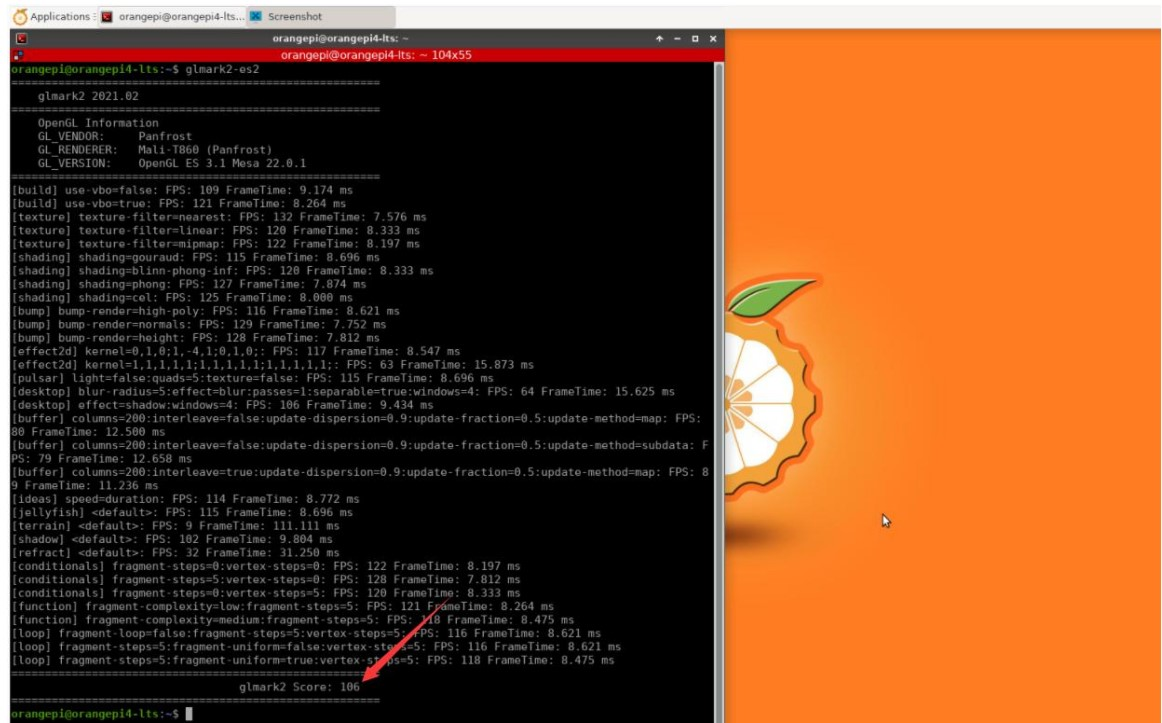## 使用高通的Snapdragon 820启用深度相机

使用神经网络解决方案（图 1），我们可以使用 HVX 模块同时对两个相机图像进行边缘检测过程。一....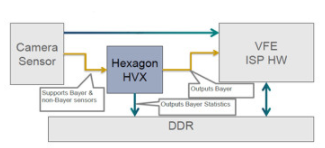小马智行将成为首批基于NVIDIA DRIVE Hyperion 计算架构及DRIVE Orin 系....Pony.ai小马智行 发表于 06-22 15:22 135次 阅读

## AresDB基于GPU运算的实时分析存储引擎和查询引擎

./oschina_soft/aresdb.zip
发表于 06-22 15:06 10次 阅读## NVIDIA为天壤提供计算能力强劲AI平台和行业解决方案

天壤成立于 2016 年，是一家专注于通用智能研究的创新企业。致力于打造复杂系统下的通用人工智能平台....科技绿洲 发表于 06-22 11:25 373次 阅读

## NVIDIA 的CUDA平台能够助力实现卓越游戏体验

越来越多的人在使用 NVIDIA 产品。机器人专家、视觉艺术专家、数据科学家等各类创新者和创作者都信....科技绿洲 发表于 06-22 10:55 173次 阅读

## NVIDIA技术助力各个领域加快创新速度

更多问题，更多可能：NVIDIA 技术助力人类为之努力的各个领域加快创新速度。

## 得心应手的AI：NVIDIA Research助力内容创作者使用3D物体进行即兴创作

可逆渲染流程 NVIDIA 3D MoMa 将于本周在新奥尔良举行的计算机视觉和模式识别会议 CVP....Blue5 发表于 06-22 10:27 1047次 阅读## NVIDIA助力集度ROBO-01概念车发布

电动汽车制造商集度展示了未来汽车的交互式 AI 驾驶体验。

## NVIDIA助力天壤推出全新蛋白质设计平台

天壤成立于 2016 年，是一家专注于通用智能研究的创新企业。致力于打造复杂系统下的通用人工智能平台....

## Imagination推出32位嵌入式解决方案IMG RTXM-2200

Imagination Technologies宣布推出其首款实时嵌入式IMG RISC-V CPU....科技绿洲 发表于 06-22 10:07 211次 阅读

## 使用GPU加速RELION进行生物结构解析

NVIDIA 与智东西公开课共同策划推出「GPU 加速高性能计算（HPC）经典应用在线研讨会」。研讨....

## NVIDIA Aerial SDK的概念及特性

构建和部署 GPU 加速的 5G 虚拟无线接入网 (vRAN)。

## NVIDIA Jetson AGX Orin开发套件开箱

NVIDIA 4 月份推出了新一代嵌入式开发套件JetsonAGX Orin，这是目前市场上性能强大....

## 使用NVIDIA QAT工具包实现TensorRT量化网络的设计

目前， TensorFlow 在其开源软件 模型优化工具包 中提供非对称量化。他们的量化方法包括....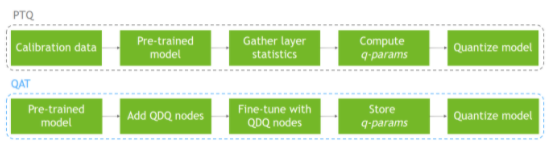## 改进VPI和PyTorch之间的互操作性

创建一个名为PyTorchDetection的类来处理所有 PyTorch 对象和调用。创建此类....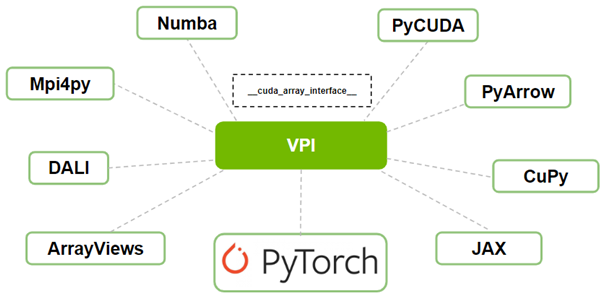## 最大限度地减少Jetson上的存储使用

NVIDIA Jetson 为开发提供了灵活的存储选项/配置，但一些 Jetson 模块配备了有限的....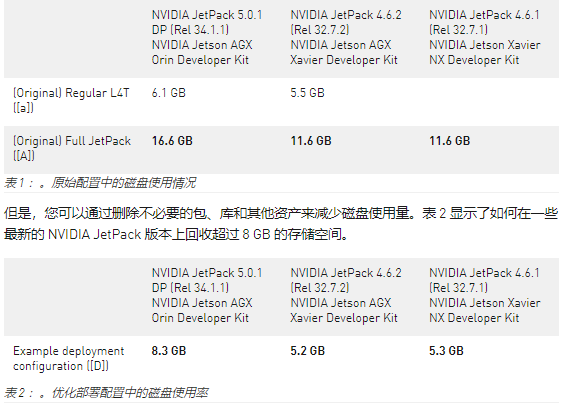## 英特尔宣布面向台式机的首款A3系列显卡面市

全新英特尔锐炫 A380显卡为主流游戏玩家和内容创作者提供新一代技术。英特尔中国 发表于 06-21 16:11 436次 阅读

## 让AI应用程序为本机云做好准备

Fleet Command 将应用程序部署为容器。通过使用容器，您可以在同一系统上部署多个应用程....

## 使用ARIA Cybersecurity和NVIDIA实时阻止现代安全攻击

对于即将推出的增强功能 NVIDIA Morpheus 应用框架 将使用高级 ML 和 AI 算....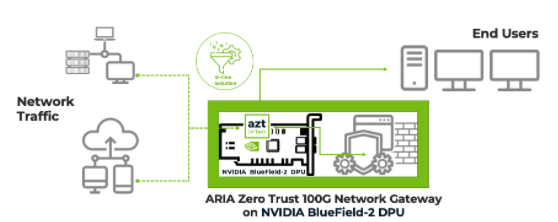## 使用TREX探索NVIDIA TensorRT引擎

元数据 JSON 文件描述了引擎的生成器配置以及用于构建引擎的 GPU 的相关信息。此信息为引擎....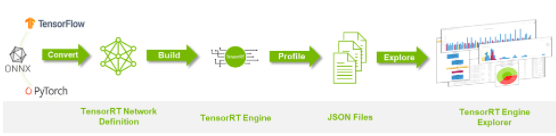## 提高Kubernetes的GPU利用率

在处理多个 CUDA 应用程序时，每个应用程序都可能没有充分利用 GPU 的资源，您可以使用简单....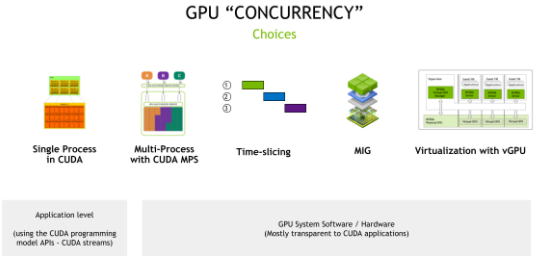## 使用最新的TAO工具包简化AI模型开发

NVIDIA AI 企业 提供了对 TAO 工具包的企业支持，这是一个用于 AI 开发和部署的端....

## 摩尔线程与迈动互联致力于为客户打造智能运维管控解决方案

摩尔线程MTT S系列GPU与迈动互联IBMS智慧建筑可视运维平台近日顺利完成兼容性适配。测试结果显....科技绿洲 发表于 06-21 15:28 171次 阅读

## 使用NVIDIA Clara Parabricks 3.8加速基因组分析

与基于CPU的环境相比，最先进的生物信息学工具的速度提高了60倍。全基因组工作流程的端到端分析只....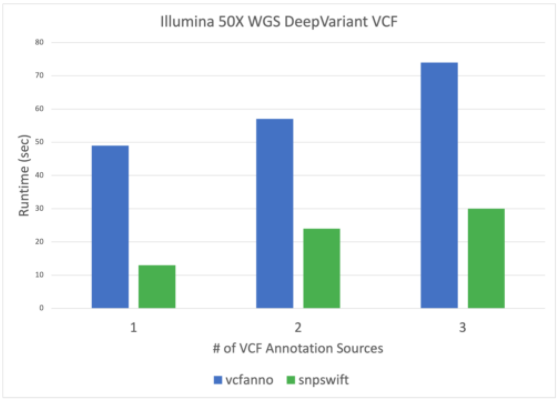## 用AI和高性能计算应对边缘数据挑战

虽然我们的许多突出的 edge HPC 应用程序都专注于流式视频和成像管道，但 NVIDIA H....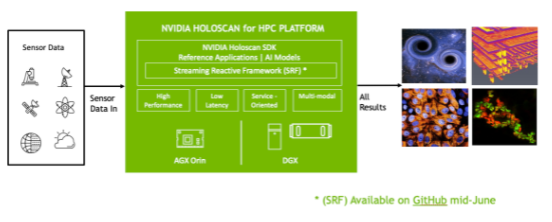## 了解何时使用DOCA驱动程序和DOCA库

DOCA 简化了 BlueField DPU 的编程和应用程序开发，并通过提供更高级别的抽象消除了障....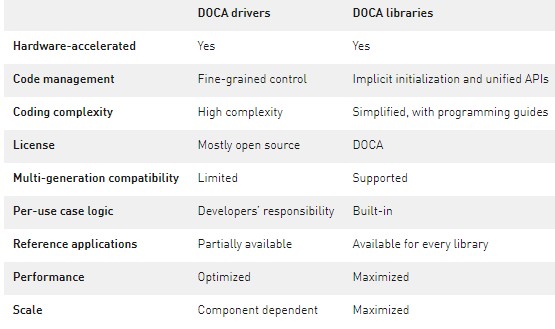## 通过全栈创新推动高性能计算

A100 还以单处理器的形式出现在操作系统中，只需要启动一个 MPI 列即可充分利用其性能。而且....## 请问在RK3399pro中间计算时能否调用GPU的一些现成数据库或函数来计算

我在用RK3399pro的NPU做深度学习计算，使用了两个rknn模型进行推理，但是在这两个rknn模型之间需要进行一些中间计算（从第一...
发表于 05-09 15:26 3558次 阅读

## tf代码为什么在rk3399上训练比没有gpu的电脑慢很多

把tf代码从电脑上放到rk3399上运行训练，比电脑慢的很多很多，板子不是带有npu吗？是不是代码需要改动什么啊？ ...
发表于 04-22 16:23 8554次 阅读

## 简单介绍下Arm Mali的GPU系列

对于手机终端来说，GPU图像处理能力是衡量一台手机的性能标杆。首先，是UI流畅性，大家拿到手机都得先划来划去看下UI是否...
发表于 04-12 11:01 4551次 阅读

## 充分利用Arm NN进行GPU推理

Arm拥有跨所有处理器的计算IP。而且，无论您要在GPU，CPU还是NPU上进行ML推理，都可以在一个通用框架下使用它们：...
发表于 04-11 17:33 4104次 阅读

## 介绍android下的OpenCL开发步骤

1、介绍android下OpenCL开发 Firefly rk3288采用Mali-T764的GPU，该GPU支持OpeCL 1.1。下面一步一步介绍android...
发表于 04-11 14:35 2429次 阅读

## AIO-3288C常用命令资料合集

1、CPU，DDR，GPU频率测量 当我们拿到 AIO-3288C 的机器时候，想看一下机器当前 CPU，DDR，GPU 跑的是什...
发表于 04-07 18:00 3696次 阅读

## 英伟达DPU的过“芯”之处

最近几年，经常关注科技圈的朋友们总会发现，每次遇到厂商有重大发布，就总能看到“颠覆”、“极致”、“革命性”等概念出现在发...
发表于 03-29 14:42 2785次 阅读

## Intel即将推出的GPU将与Arm兼容

英特尔正在使其GPU多样化，以与x86以外架构的处理器兼容，这可能是使其从芯片制造商成为以代工为首要业务的公司的一步。 英特...
发表于 03-29 14:41 2643次 阅读

## Arm Neoverse NVIDIA Grace CPU 超级芯片：为人工智能的未来设定步伐

人工智能 (AI) 将改变世界。但要实现其潜力，我们将不得不改变设计计算系统的方式。 诸如在云中训练神经网络或在边缘实时执...
发表于 03-29 14:40 2832次 阅读